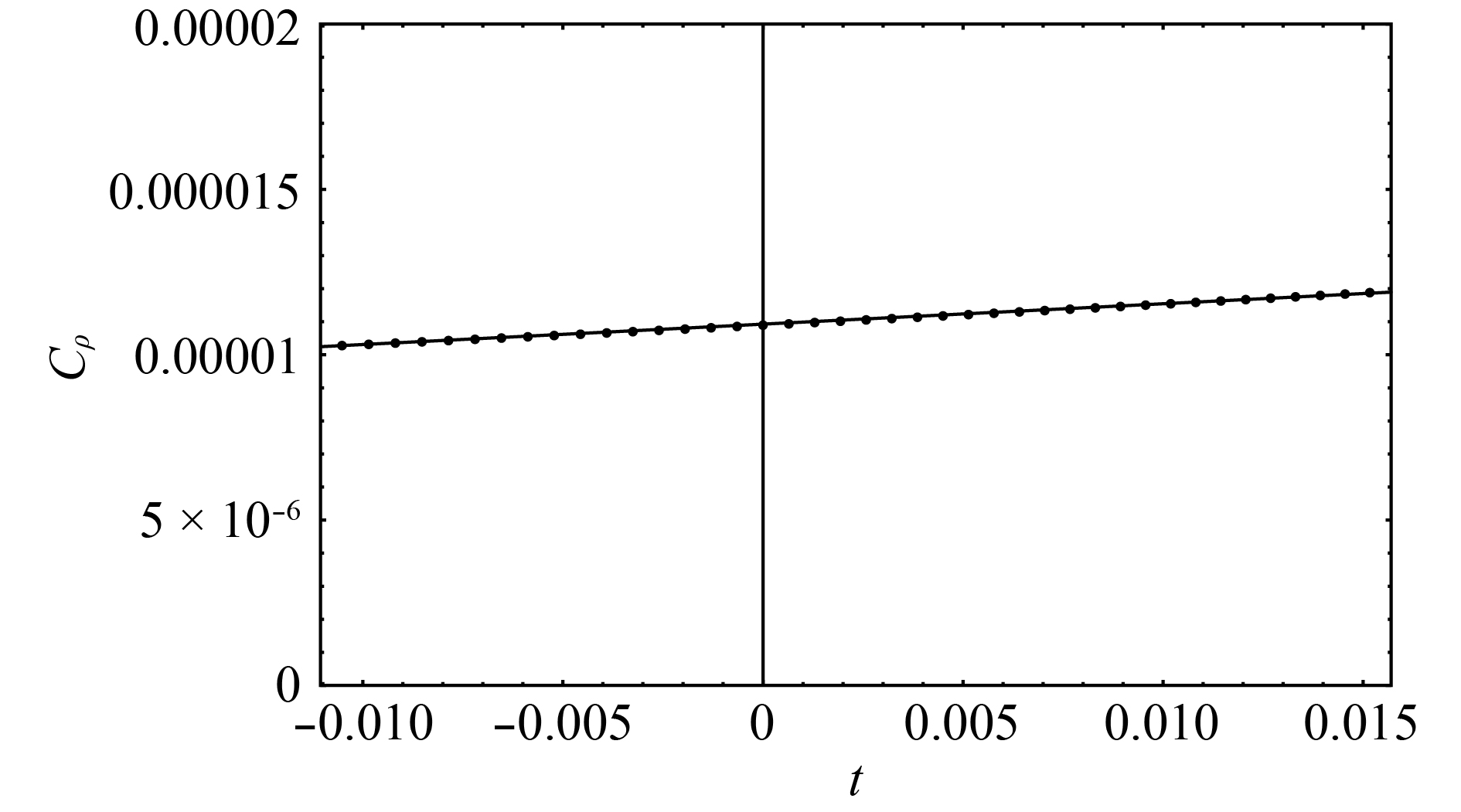# Criticality of QCD in a holographic QCD model with critical end point• The thermodynamics of strongly interacting matter near the critical end point are investigated in a holographic QCD model, which can describe the QCD phase diagram in $T-\mu$plane qualitatively. Critical exponents along different axes ( $\alpha,\beta,\gamma,\delta$) are extracted numerically. It is given that $\alpha\approx 0$, $\beta\approx 0.54$, $\gamma \approx 1.04$, and $\delta \approx 2.97$, which is similar to the three-dimensional Ising mean-field approximation and previous holographic QCD model calculations. We also discuss the possibilities to go beyond the mean field approximation by including the full back-reaction of the chiral dynamics in the holographic framework.
••Get Citation
Xun Chen, Danning Li and Mei Huang. Criticality of QCD in a holographic QCD model with critical end point[J]. Chinese Physics C, 2019, 43(2): 1-1. doi: 10.1088/1674-1137/43/2/023105
Xun Chen, Danning Li and Mei Huang. Criticality of QCD in a holographic QCD model with critical end point[J]. Chinese Physics C, 2019, 43(2): 1-1.Milestone
Received: 2018-10-18
Article Metric

Article Views(269)
PDF Downloads(22)
Cited by(0)
Policy on re-use
To reuse of Open Access content published by CPC, for content published under the terms of the Creative Commons Attribution 3.0 license (“CC CY”), the users don’t need to request permission to copy, distribute and display the final published version of the article and to create derivative works, subject to appropriate attribution.
###### 通讯作者: 陈斌, bchen63@163.com
• 1.

沈阳化工大学材料科学与工程学院 沈阳 110142

Title:
Email:

## Criticality of QCD in a holographic QCD model with critical end point

• 1. Central China Normal University, Wuhan 430079, China
• 2. Institute of High Energy Physics, Chinese Academy of Sciences, Beijing 100049, China
• 3. Department of Physics and Siyuan Laboratory, Jinan University, Guangzhou 510632, China
• 4. University of Chinese Academy of Sciences, Beijing 100049, China
• 5. Theoretical Physics Center for Science Facilities, Chinese Academy of Sciences, Beijing 100049, China

Abstract: The thermodynamics of strongly interacting matter near the critical end point are investigated in a holographic QCD model, which can describe the QCD phase diagram in $T-\mu$plane qualitatively. Critical exponents along different axes ( $\alpha,\beta,\gamma,\delta$) are extracted numerically. It is given that $\alpha\approx 0$, $\beta\approx 0.54$, $\gamma \approx 1.04$, and $\delta \approx 2.97$, which is similar to the three-dimensional Ising mean-field approximation and previous holographic QCD model calculations. We also discuss the possibilities to go beyond the mean field approximation by including the full back-reaction of the chiral dynamics in the holographic framework.

### HTML1.   Introduction2.   Einstein-Maxwell-Dilaton system and CEP of QCD phase diagram3.   Holographic results of critical exponents at CEP
Reference (89)
PDF查看关注分享

Top

### 目录/DownLoad:  Full-Size Img  PowerPoint